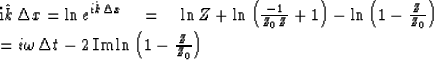# CAUSALITY AND WAVE PROPAGATION

The principle of causality, i.e., no response before a stimulus, places certain restraints upon the nature of wave propagation. Causality often seems to be violated because of

1.
Approximations in a theory which are made to simplify it.
2.
Fitting inappropriate curves to experimental observations.

3.
Approximations made in converting differential equations to difference equations.

Violations of causality often result from seemingly inconsequential approximations at spectral frequencies outside the range of practical interest. The seemingly inconsequential approximations often take on significance because of the very weak convergence in the Hilbert transform. With regard to (1) and (2), the violation of causality may turn out to be a low price to pay compared with the cost of a more precise analysis. With regard to (3) or computation in general, the violation of causality usually has disastrous consequences. Even though difference equations may have proper behavior at frequencies of interest, exponential growth at other frequencies is almost always so severe as to completely obliterate the desired solution.

Most wave-propagation theories are worked out in the frequency domain in homogeneous materials. If they are further done in cartesian geometry, the end result is that propagation along the x axis is given by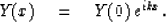(23)
What the wave theory provides is an expression for k in terms of frequency and all the physical parameters such as velocity, viscosity, etc. We will now take an after-the-derivation'' look at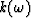and see if it satisfies causality. In other words, (23) is like the filter situation depicted in Figure 7. Y(0) is the filter input, Y(x) is like the output, and eikx is a filter which models the effect of propagation. The question is whether the filters are one-sided. This depends upon the detailed form of.

 3-7 Figure 7 Modeling wave propagation with filters.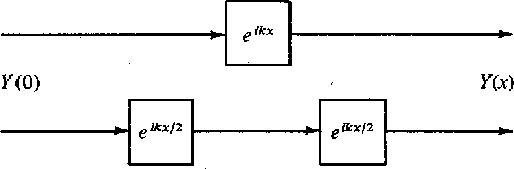First, consider the simple case whenis real for all real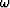.Then eikx is an all-pass filter. It is realizable if and only if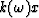is a monotonically increasing function of.

Next, supposeis complex. This means that attenuation will occur. Clearly energy conservation requires that the real part of ikx be negative.

Define P(Z) to be a realizable all-pass filter and B(Z) to be a realizable minimum-phase wavelet. Any realizable function can be represented in the form B(Z) P(Z). We will require eikx to be so represented.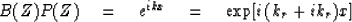(24)
Taking logs and using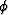to denote phase, we have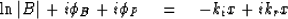(25)
Splitting into real and imaginary parts we obtain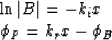First of all, from the attenuation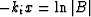we can by Hilbert transform compute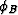.When it is subtracted from kr x we are left with the phase shift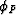of the all-pass filter. Recall from Sec. 2-6 (on all-pass filters) that if and only ifis monotonically increasing, we have a causal all-pass filter. In conclusion, the test of causality for a functionlies in computing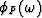and seeing whether it is monotonically increasing for all frequencies.

A great deal of confusion results from attenuation laws which are thought to be of the form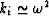(gaussian attenuation) or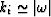(sometimes called constant Q). The reason is that these functions are not integrable so they do not have convergent Hilbert transforms; that is, theturns out to be infinite. These functions correspond to time pulses like the gaussian time function e-t2 which do not have a time before which they are zero. Such functions cannot strictly be considered to be associated with any causal process; however, they may give excellent practical approximations. In reality, physical dissipation is generally associated with a relaxation phenomenon having a finite relaxation time. For frequency values below the relaxation value, the attenuation may appear to be increasing indefinitely with frequency, but, in fact, the attenuation decreases above the characteristic relaxation frequency. The attenuation also provides a phase velocity aberration because of the addition oftobut this is generally considered to be too small to measure.

Now let us take up an example of computer modeling of wave propagation. The simplest example is propagation without dissipation. Then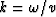where v is the velocity. Rather than attempt to construct a filter which will carry waves a long distance we will only attempt to carry them a distance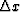.The filter may be used N times to propagate a distance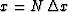.We will use the bilinear truncation of the power series for exponential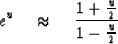(26)
and the bilinear representation for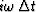, namely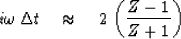(27)
With these andand the definition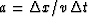we obtain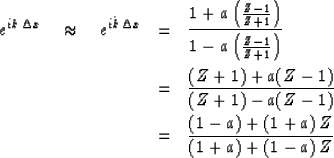(28)
That this is the general form of an all-pass filter may be seen by taking Z0 = -(1 + a)/(1 -a). Then (28) becomes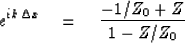(29)
The denominator will be minimum phase and the all-pass filter will be realizable if Z0 > 1 or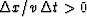.This means that present and past values of the inputs will determine the output if the wave is being projected in the +x direction. If it is desired to project the wave backwards in space (negative), then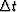must be taken negative, so that present and future input values determine the present output.

## EXERCISES:

1. What is the velocity error as a function offor the filter of (28) for values of a = .1, 1., and 2. HINT:   Let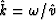so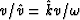.Then note that